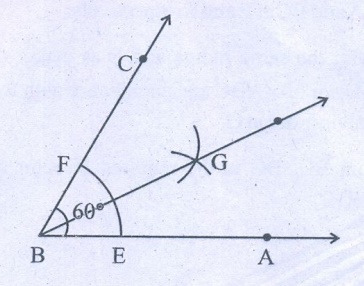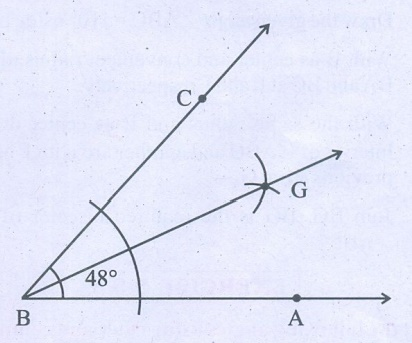Home | | Maths 7th Std | Exercise 5.4 (Construction of angle bisector of an angle)

# Exercise 5.4 (Construction of angle bisector of an angle)

7th Maths : Term 1 Unit 5 : Geometry : Construction of angle bisector of an angle : Text Book Back Exercises Questions with Answers, Solution : Construct the following angles using protractor and draw a bisector to each of the angle using ruler and compass.

Exercise 5.4

1. Construct the following angles using protractor and draw a bisector to each of the angle using ruler and compass.

(a) 60°

(b) 100°

(c) 90°

(d) 48°

(e) 110°

(a) 60oStep 1 : Draw the given angle ABC = 60° using protractor.

Step 2 : With B as center and convenient radius, draw an arc to cut BA and BC at E and F respectively.

Step 3 : With the same radius and E as center draw an arc in the interior of ABC and another arc with F as center to cut the previous arc at G.

Step 4 : Join BG. BG is the required bisector of the given angle ABC.

(b) 100°Step 1 : Draw the given angle ABC =100° using protractor.

Step 2 : With B as center and convenient radius, draw an arc to cut BA and BC at E and F respectively.

Step 3 : With the same radius and E as center draw an arc in the interior of ABC and another arc with F as center to cut the previous arc at G.

Step 4 : Join BG. BG is the required bisector of the given angle ABC.

(c) 90°Step 1 : Draw the given angle ABC = 90° using protractor.

Step 2 : With B as center and convenient radius, draw an arc to cut BA and BC at E and F respectively.

Step 3 : With the same radius and E as center draw an arc in the interior of ABC and another arc with F as center to cut the previous arc at G.

Step 4 : Join BG. BG is the required bisector of the given angle ABC.

(d) 48°Step 1 : Draw the given angle ABC = 48° using protractor.

Step 2 : With B as center and convenient radius, draw an arc to cut BA and BC at E and F respectively.

Step 3 : With the same radius and E as center draw an arc in the interior of ABC and another arc with F as center to cut the previous arc at G.

Step 4 : Join BG. BG is the required bisector of the given angle ABC.

(e) 110°Step 1 : Draw the given angle ABC =110° using protractor.

Step 2 : With B as center and convenient radius, draw an arc to cut BA and BC at E and F respectively.

Step 3 : With the same radius and E as center draw an arc in the interior of ABC and another arc with F as center to cut the previous arc at G.

Step 4 : Join BG. BG is the required bisector of the given angle ABC.

Tags : Questions with Answers, Solution | Geometry | Term 1 Chapter 5 | 7th Maths , 7th Maths : Term 1 Unit 5 : Geometry
Study Material, Lecturing Notes, Assignment, Reference, Wiki description explanation, brief detail
7th Maths : Term 1 Unit 5 : Geometry : Exercise 5.4 (Construction of angle bisector of an angle) | Questions with Answers, Solution | Geometry | Term 1 Chapter 5 | 7th Maths